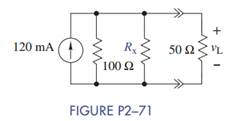Chat Now

# Select a value of R x in Figure P2−71 so that v L = 2 V. Repeat for 4 V and 6 V. Caution: R x..

### Select a value of R x in Figure P2−71 so that v L = 2 V. Repeat for 4 V and 6 V. Caution: R x..

Select a value of Rx in Figure P2−71 so that vL = 2 V. Repeat for 4 V and 6 V. Caution: Rmust be positive.manish jayant 28-Jul-2020Get solution